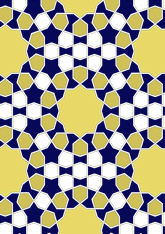data156/F27

## Geometry

• The symmetry group of the tiling is 2*22 (cmm).
• All the internal angles of the constituent polygons are a multiple of 18°.
• Contains one regular five-pointed star polygon with vertex angle of 72°.
• Contains one regular 10-pointed star polygon with vertex angle of 108°.
• There are five non-regular reflective tiles.
• The tiling satisfies the interlace condition and has three finite interlaces and no infinite interlace with straight cross-overs.
• The tiling is edge-to-edge.
• As drawn, contains about 290 polygons.

## References

Publications referenced: